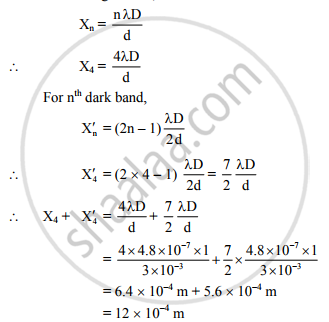# In a Biprism Experiment, a Slit is Illuminated by a Light of Wavelength 4800 A The Distance Between the Slit and Biprism is 15 Cm and the Distance Between the Biprism and Eyepiece is 85 Cm. - Physics

In a biprism experiment, a slit is illuminated by a light of wavelength 4800 Å The distance between the slit and biprism is 15 cm and the distance between the biprism and eyepiece is 85 cm. If the distance between virtual sources is 3 mm, determine the distance between 4th bright band on one side and 4th dark band on the other side of the central bright band.

#### Solution

λ = 4800 Å = 4.8 × 10−7m,

d = 3 mm = 3 × 10−3m,

D = 15 + 85 = 100 cm = 1 m

Distance between 4th bright band on one side and 4th dark band on the other side of the central
bright band, (X4 + X′4) = ?
For nth bright band,The distance between 4th bright band on one side and 4th dark band on the other side of the central bright band is 12 × 10−4 m.

Concept: Measurement of Wavelength by Biprism Experiment
Is there an error in this question or solution?
2013-2014 (October)

Share